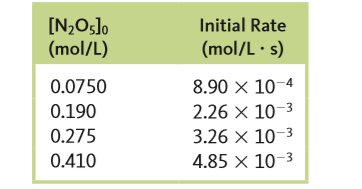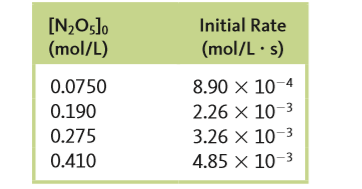# Problem: The following data were obtained for the gas‑phase decomposition of dinitrogen pentoxide,2 N2O5 (g) → 4 NO2 (g) + O2 (g)Defining the rate as -Δ[N2O5] / Δt, write the rate law and calculate the value of the rate constant.

###### FREE Expert Solution

Recall that the rate law only focuses on the reactant concentrations and has a general form of:

k = rate constant
A & B = reactants
x & y = reactant orders

Step 1. Calculate the order of the reaction with respect to N2O5.

Use experimental data 1 and 2 (you can use any):x = order

(Larger concentration should be on the numerator)

Solve for x:

$\frac{\mathbf{2}\mathbf{.}\mathbf{26}\mathbf{×}{\mathbf{10}}^{\mathbf{-}\mathbf{3}}}{\mathbf{8}\mathbf{.}\mathbf{90}\mathbf{×}{\mathbf{10}}^{\mathbf{-}\mathbf{4}}}\mathbf{=}\frac{{\left[\mathbf{0}\mathbf{.}\mathbf{190}\right]}^{\mathbf{x}}}{{\left[\mathbf{0}\mathbf{.}\mathbf{0750}\right]}^{\mathbf{x}}}$

since the numerator and the denominator are raised to the same power, you can simplify the equation to:

$\frac{\mathbf{2}\mathbf{.}\mathbf{26}\mathbf{×}{\mathbf{10}}^{\mathbf{-}\mathbf{3}}}{\mathbf{8}\mathbf{.}\mathbf{90}\mathbf{×}{\mathbf{10}}^{\mathbf{-}\mathbf{4}}}\mathbf{=}{\left[\frac{\mathbf{0}\mathbf{.}\mathbf{190}}{\mathbf{0}\mathbf{.}\mathbf{0750}}\right]}^{\mathbf{x}}\phantom{\rule{0ex}{0ex}}\mathbf{2}\mathbf{.}\mathbf{53}\mathbf{=}{\left[\mathbf{2}\mathbf{.}\mathbf{53}\right]}^{\mathbf{x}}$

2.53 raised to the power of 1 = 2.53

x = 1 → 1st order with respect to N2O5

Step 2. Determine the rate law of the reaction

Substitute N2O5 and O2 and their orders:

rate law = k[N2O5]1

or simply

rate = k[N2O5]

Step 3. Calculate the value of the rate constant

96% (105 ratings)###### Problem Details

The following data were obtained for the gas‑phase decomposition of dinitrogen pentoxide,

2 N2O(g) → 4 NO2 (g) + O(g)Defining the rate as -Δ[N2O5] / Δt, write the rate law and calculate the value of the rate constant.

Frequently Asked Questions

What scientific concept do you need to know in order to solve this problem?

Our tutors have indicated that to solve this problem you will need to apply the Rate Law concept. You can view video lessons to learn Rate Law. Or if you need more Rate Law practice, you can also practice Rate Law practice problems.

What professor is this problem relevant for?

Based on our data, we think this problem is relevant for Professor Demesa's class at BAYLOR.

What textbook is this problem found in?

Our data indicates that this problem or a close variation was asked in Chemistry: An Atoms First Approach - Zumdahl Atoms 1st 2nd Edition. You can also practice Chemistry: An Atoms First Approach - Zumdahl Atoms 1st 2nd Edition practice problems.